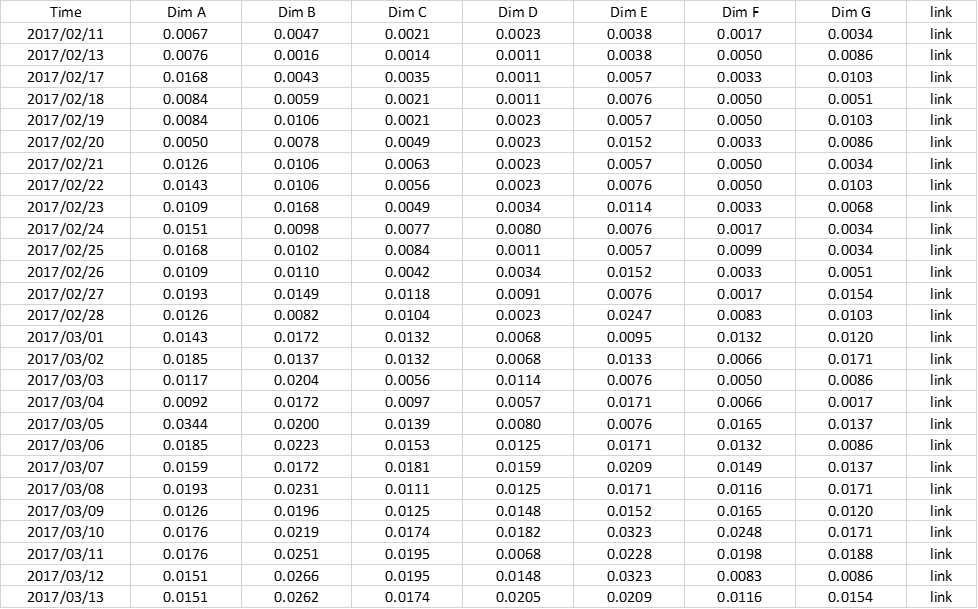# How to Create the Joy Plot

Today I'll write a note on my small research: How to create the Joy Plot.

References are here.

https://thedataism.wordpress.com/2017/07/22/joy-plots-in-tableau/

https://public.tableau.com/profile/ken.flerlage#!/vizhome/JoyPlotTemplateWithDatesonAxis/Dashboard

https://public.tableau.com/profile/yoshitaka6076#!/vizhome/InProgressJoyPlot/Dashboard2?publish=yesWhy the viz is called "JOY PLOT"? According to the first reference, it's named after a music album "UNKNOWN PLEASURES" played by "JOY DIVISION".The data we'll use for the plot is like this:In the data, we have Time and some measures where column names are from Dim A to Dim G.

The joy plot requires to do a data manipulation like a Sankey Chart so we need the "link" column. And we need this small table to JOIN.Then we JOIN them in Tableau.After we JOIN this we pivot the data like this.The preparation is done. Let's move forward.

Since we duplicated the value by JOINing the data, we need to adjust the value with the caluculation here:

CASE [Purpose] WHEN "Primary" THEN [Value] ELSE 0 END

And for arranging the values with the plots, we need the following calculation.

Key

CASE [Dimension] WHEN "Dim A" THEN [Position A] WHEN "Dim B" THEN [Position B] WHEN "Dim C" THEN [Position C] WHEN "Dim D" THEN [Position D] WHEN "Dim E" THEN [Position E] WHEN "Dim F" THEN [Position F] ELSE [Position G] END

The [Position A] to [Position F] are parameters that I made and they have an integer from 1 to 7.

And to put all seven different values belonging to the different seven dimensions, we need this calculation here.

[Value Adjusted] + ({FIXED:COUNTD([Dimension])} - [Key] + 1)*[Spacing]

The [Spacing] is also a parameter to "Space" the values.

Lastly, since we're going to use Polygon Marks, we need to specify the path of how to connect the polygons.

Path

IF [Purpose]="Primary" THEN [Time] ELSE DATE((INT({FIXED [Dimension]: MAX([Time])})*2) - INT([Time]) + 1) END

It would be easy to see how the "Path" works along "Time" column.As you can see here, if the "purpose" is "Primary", the Path is increasing as the Time goes.

If the "purpose" is "Zero", the path is increasing as the Time goes back. It's how the path work.

So basically the path is turning around. When it hits the corner, the purpose changes from Primary to Zero, then the path goes back as it increase.

And then you can put everything like this. One small tip is here: I sort the dimension with "Key", which allows me to arrange the order in the color legend, based on the number (or rank) of the Position parameters. You can try it with and without the sorting and see how the color legend can be sorted dynamically.Then it's done. In conclusion the Joy Plot requires only several steps.

1. Data Manipulation: JOIN and pivot.

2. Create some calculations.

3. Put them and enjoy the Joy Plot.

I hope you enjoyed this article. Recently I started Twitter(@yoshi_dataviz) for #makeovermonday that is a social data-viz project. I hope you'll enjoy the project too and it'd be great if we would be connected on Twitter and enjoy the makeovers together.

See you soon.

#Tableau